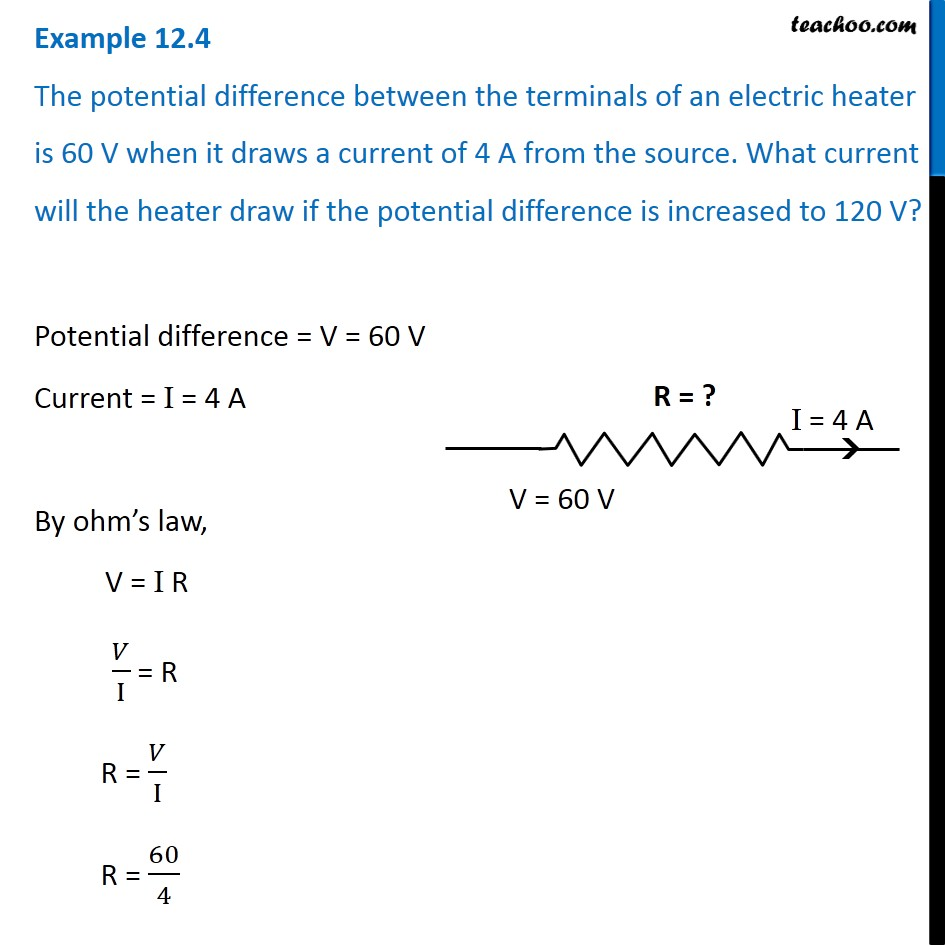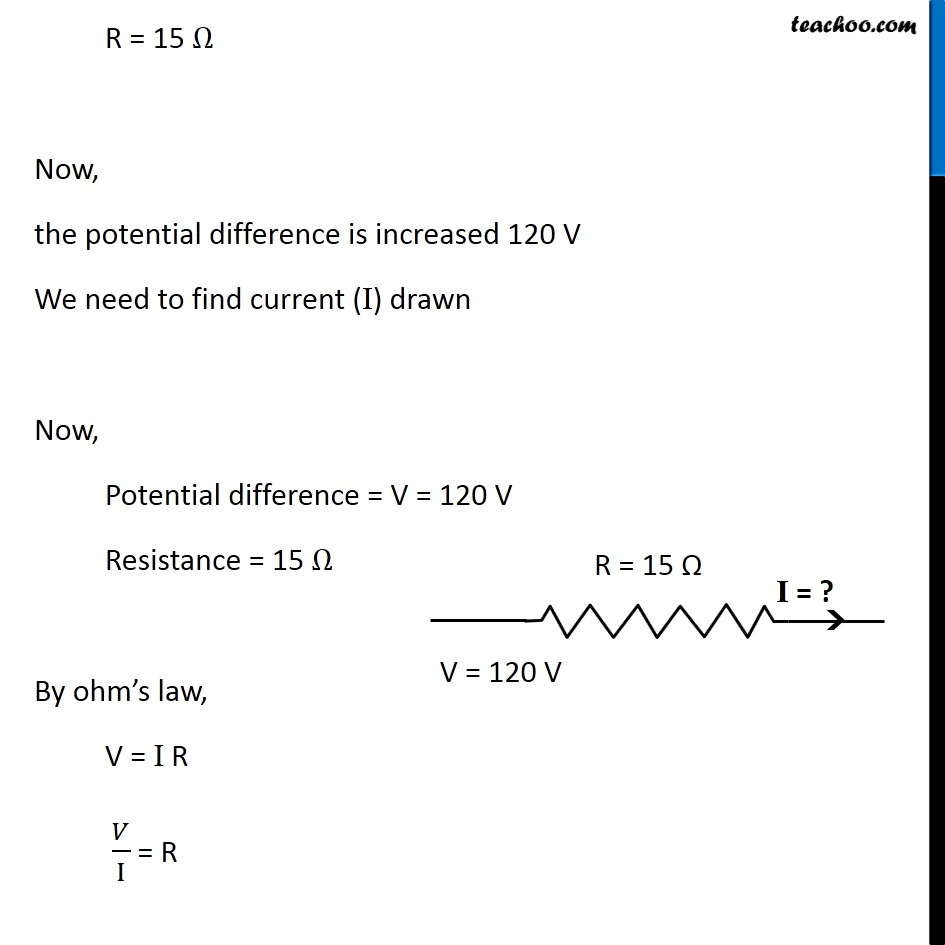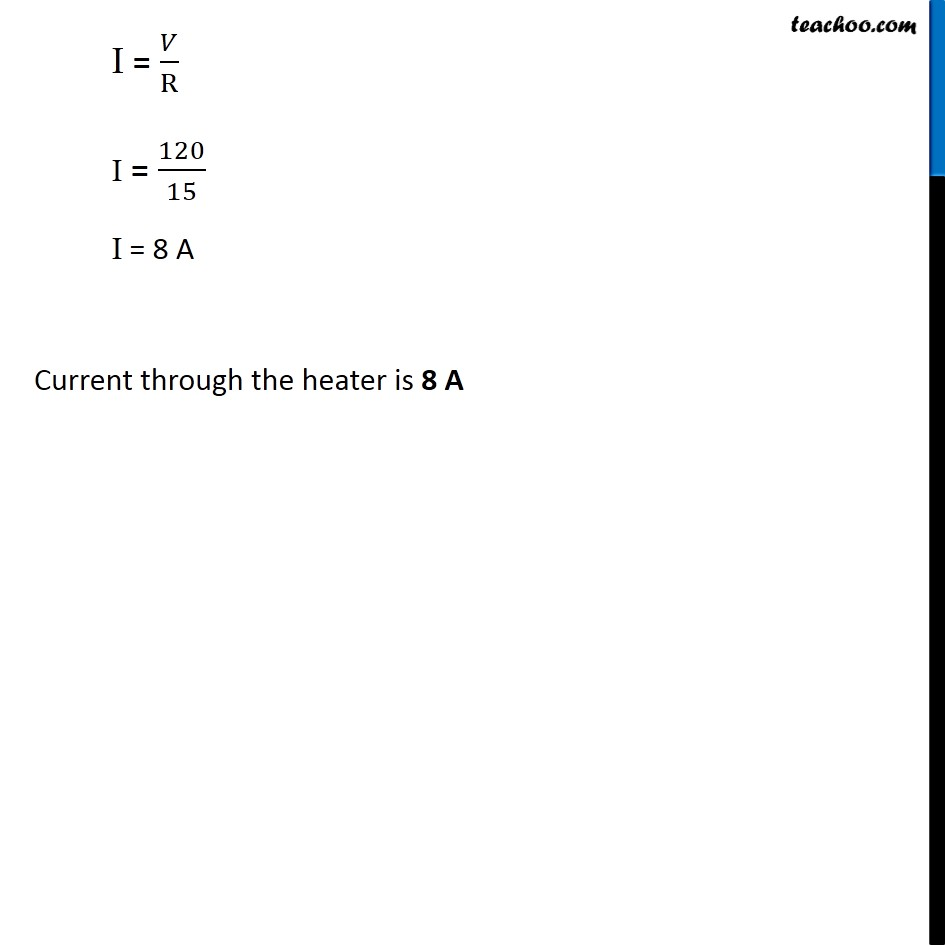Examples from NCERT Book

Class 10
Chapter 12 Class 10 - Electricity

## The potential difference between the terminals of an electric heater is 60 V when it draws a current of 4 A from the source. What current will the heater draw if the potential difference is increased to 120 V?Learn in your speed, with individual attention - Teachoo Maths 1-on-1 Class

### Transcript

Example 12.4 The potential difference between the terminals of an electric heater is 60 V when it draws a current of 4 A from the source. What current will the heater draw if the potential difference is increased to 120 V? Potential difference = V = 60 V Current = I = 4 A By ohm’s law, V = I R 𝑉/I = R R = 𝑉/I R = 60/4 R = 15 Ω Now, the potential difference is increased 120 V We need to find current (I) drawn Now, Potential difference = V = 120 V Resistance = 15 Ω By ohm’s law, V = I R 𝑉/I = R I = 𝑉/R I = 120/15 I = 8 A Current through the heater is 8 A